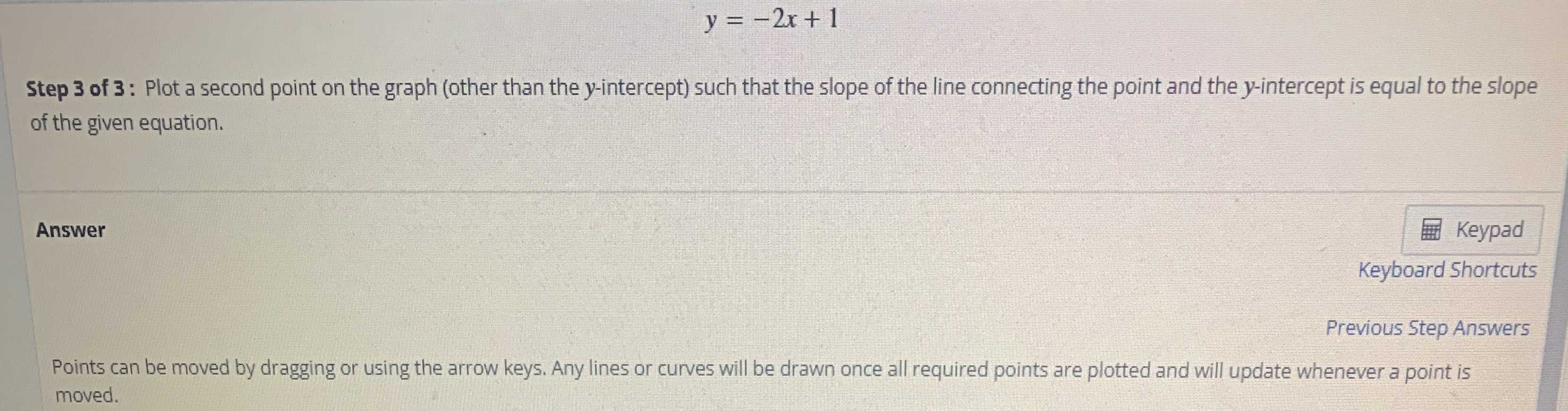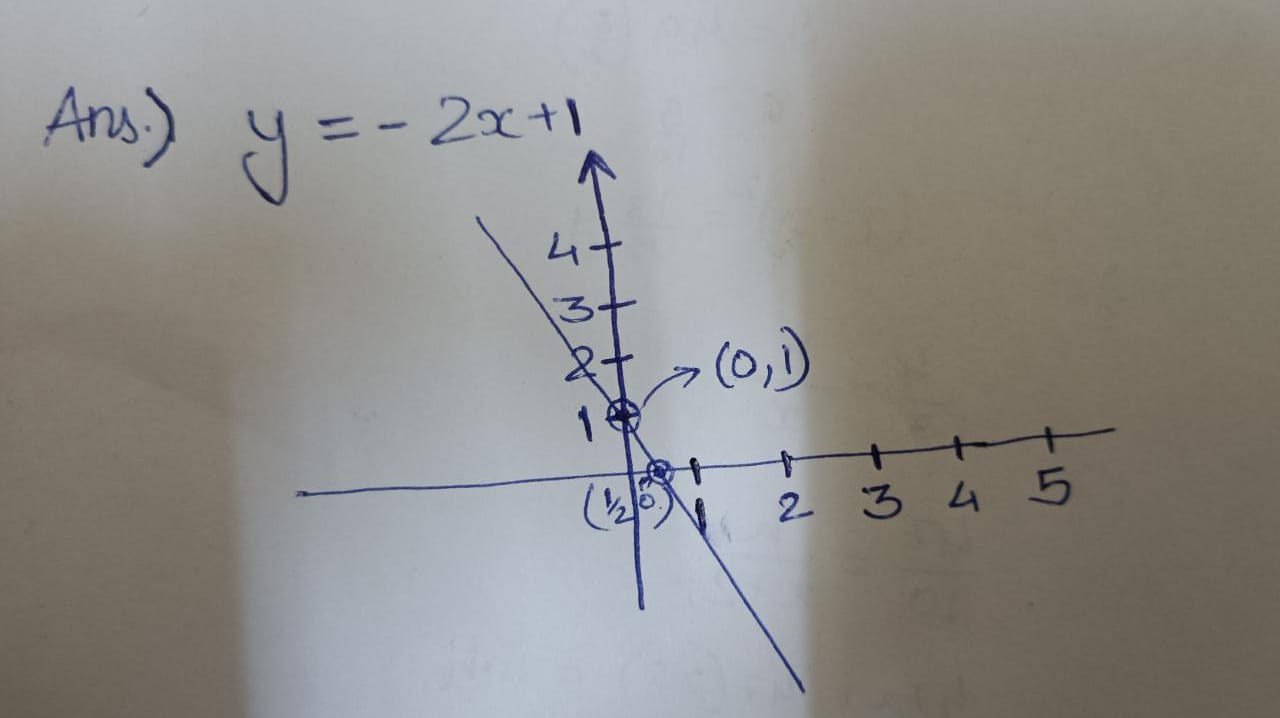### Still have math questions?

Algebra
Question$$y = - 2 x + 1$$

Step $$3$$ of $$3$$ : Plot a second point on the graph (other than the $$y$$ -intercept) such that the slope of the line connecting the point and the $$y$$ -intercept is equal to the slope of the given equation. Points can be moved by dragging or using the arrow keys. Any lines or curves will be drawn once all required points are plotted and will update whenever a point is moved.